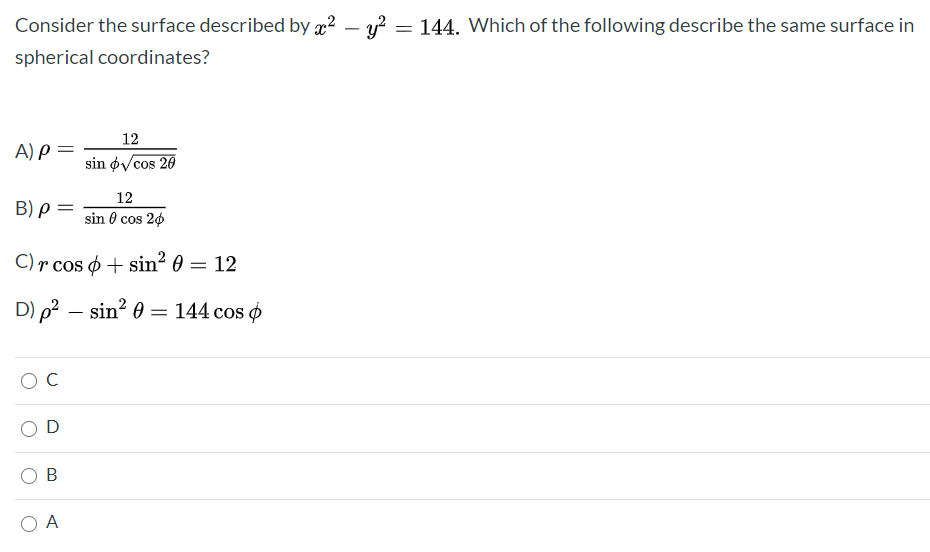# Consider the surface described by x² – v² = 144. Which of the following describe the same surface in %3D spherical coordinates? 12 A) p = sin øycos 20 12 B) p = sin 0 cos 20 C) r cos ø + sin? 0 = 12 D) p2 – sin? 0 = 144 cos ¢ -

Questionhelp_outlineImage TranscriptioncloseConsider the surface described by x² – v² = 144. Which of the following describe the same surface in %3D spherical coordinates? 12 A) p = sin øycos 20 12 B) p = sin 0 cos 20 C) r cos ø + sin? 0 = 12 D) p2 – sin? 0 = 144 cos ¢ - fullscreen

### Want to see this answer and more?

Experts are waiting 24/7 to provide step-by-step solutions in as fast as 30 minutes!*

*Response times may vary by subject and question complexity. Median response time is 34 minutes for paid subscribers and may be longer for promotional offers.
Tagged in
MathCalculus

### Other